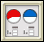Bits and Pieces II: Investigation 2: Adding and Subtracting Fractions
 Arnold WorksiteStudent Resources Danicek WorksiteStudent Resources Grimmer WorksiteStudent Resources Bits And Pieces II   Homework Help Investigation 1, ACE Exercise 19 Investigation 2, ACE Exercise 27 Investigation 3, ACE Exercise 16 Investigation 4, ACE Exercise 12 Bits And Pieces II Multiple-Choice Skills PracticeVocabulary Quiz Pearson Video Tutor Math Kids Video Adding and Subtracting FractionsCore Support Files

Decimals

Fractions

• Jamit Fractions Lessons & Games
• AAAMath Fractions, Ratios
• Illuminations Fractions
• FunSchool Action Fraction
• BBC Bitesize Fractions, Ratios
• Cool Math:
• National Library of Virtual Manipulatives
•Fraction Pieces – Work with parts and wholes to learn about fractions.Fractions - Adding – Illustrates what it means to find a common denominator and combine.Fractions - Comparing – Judge the size of fractions and plot them on a number line.Fractions - Equivalent – Illustrates relationships between equivalent fractions.Fractions - Rectangle Multiplication – Visualize and practice multiplying fractions using an area representation.
• Fraction Cafe

PercentsBits and Pieces II
Concept with Explanation
Selected Homework from ACE
Bits and Pieces II was created to help students make sense of one aspect of rational number: fraction operations. The investigations in this unit will help students to:

• Use benchmarks and other strategies to estimate the reasonableness of results of operations with fractions;
• Develop ways to model sums, differences, products, and quotients with areas, strips, and number lines;
• Use estimates and exact solutions to make decisions;
• Look for and generalize patterns in numbers;
• Use knowledge of fractions and equivalence of fractions to develop algorithms for adding, subtracting, multiplying and dividing fractions;
• Recognize when addition, subtraction, multiplication, and division is the appropriate operation to solve a problem;
• Write fact families to show the inverse relationship between addition and subtraction, and between multiplication and division;
• Solve problems using arithmetic operations on fractions; and
• Look for and generalize patterns.

 Vocabulary
 Picture Vocabuary Equivalent Fractions:  Fractions that have the same simplest form. Hundredths Grid: a 10 by 10 grid reduce: to put a fraction isn simpler form reciprocal: two numbers whose product is 1. Easily gotten by 'flipping' the numerator & denominator. i.e. 2 * 1/2 = 1 Fraction:  Part of a whole number. Written in the form of a numerator and denominator Numerator:  The part of the whole being used Denominator: The whole of the fraction.  The number of equal pieces in the whole. Common Denominator: When two fractions have the same denominator. Used for addtion and subtraction operations.